# Difference between revisions of "Size Balanced Tree"

A size balanced tree (SBT) is a self-balancing binary search tree first published by Chinese student Qifeng Chen in 2007. The tree is rebalanced by examining the sizes of each node's subtrees. Its abbreviation resulted in many nicknames given by Chinese informatics competitors, including "Sha bi" tree (Chinese: 傻屄树; pinyin: Shǎ bī shù; literally meaning "dumb cunt tree") and "Super BT", which is a homophone to the Chinese term for snot (Chinese: 鼻涕; pinyin: bítì) suggesting that it is messy to implement. Contrary to what its nicknames suggest, this data structure can be very useful, and is also known to be easy to implement. Since the only extra piece of information that needs to be stored is sizes of the nodes (instead of other "useless" fields such as weights in treaps or colours in red–black tress), this makes it very convenient to implement the select and rank operations in dynamic order statistics problems. It supports standard binary search tree operations such as insertion, deletion, and searching in O(log n) time. According to Chen's paper, "this is the fastest known advanced binary search tree to date."

## Properties

The size balanced tree examines each node's size (i.e. the number of nodes in the subtree rooted at that node) to determine when rotations should be performed. Each node$T$ in the tree satisfies the following properties:

•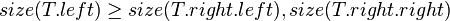$size(T.left) \ge size(T.right.left), size(T.right.right)$
•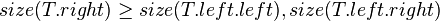$size(T.right) \ge size(T.left.left), size(T.left.right)$

In other words, each child node of$T$ is not smaller in size than the child nodes of its sibling. Clearly, we should consider the sizes of nonexistent children and siblings to be 0.

Consider the following example where$T$ is the node in question,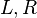$L, R$ are its child nodes, and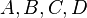$A, B, C, D$ are subtrees which also satisfy the above SBT properties on their own.

```          T
/ \
/   \
L     R
/ \   / \
A   B C   D
```

Then, the node$T$ must satisfy:

•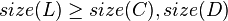$size(L) \ge size(C), size(D)$
•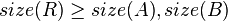$size(R) \ge size(A), size(B)$